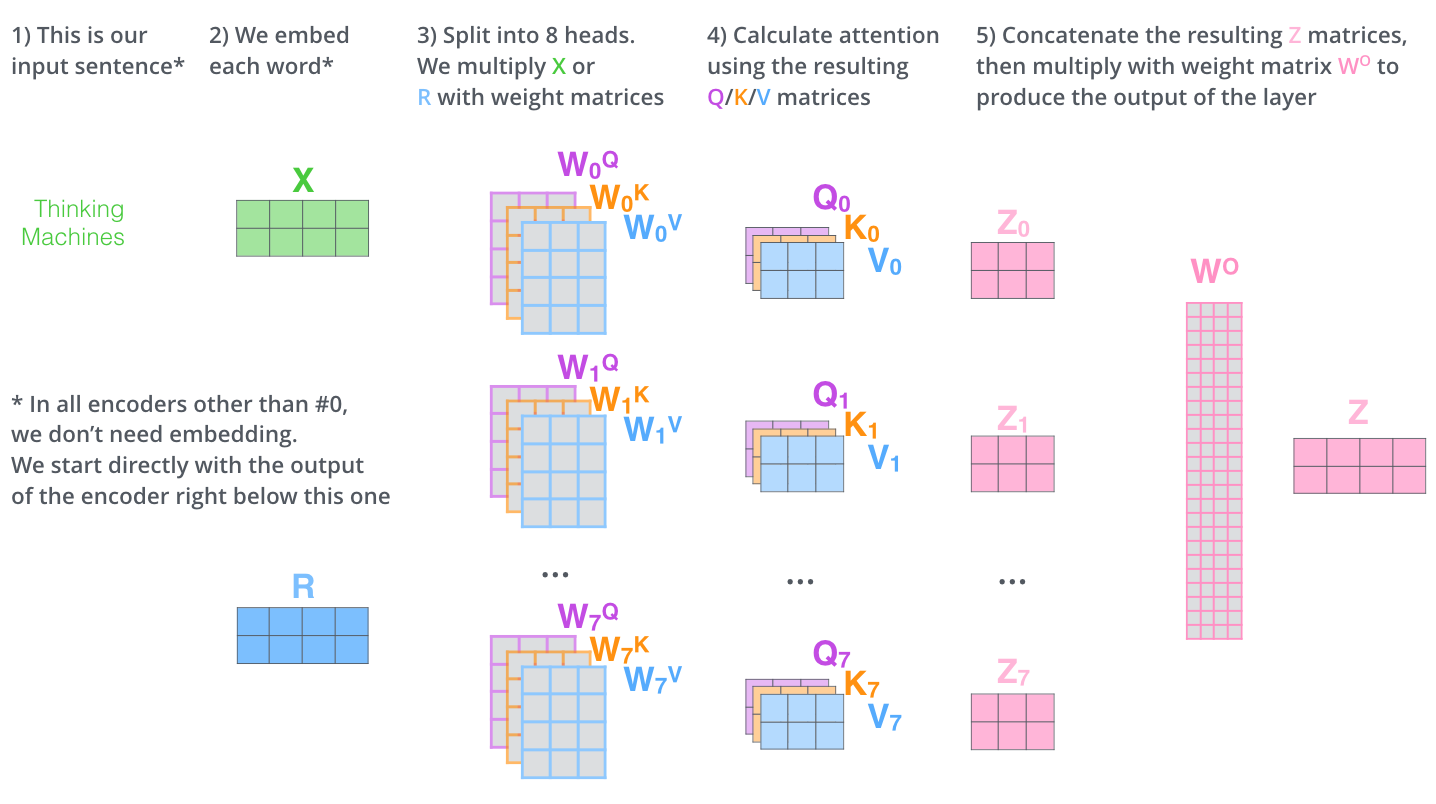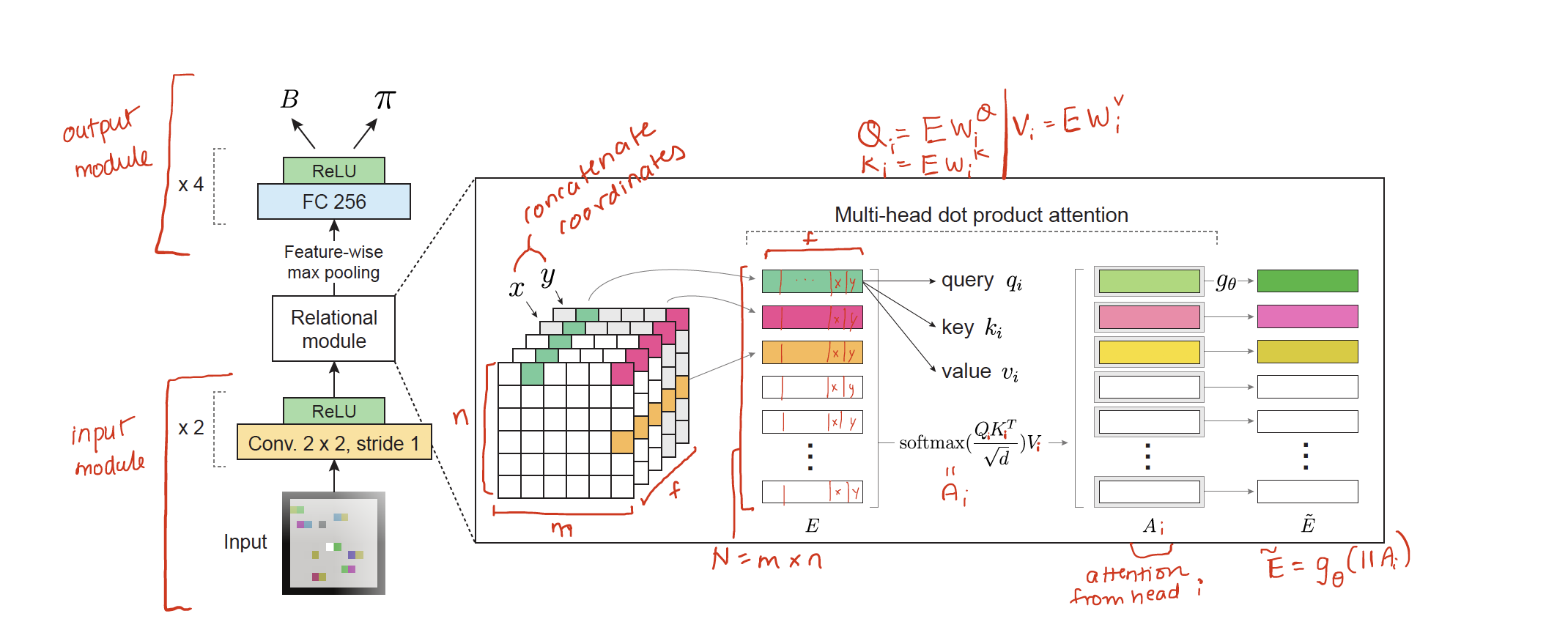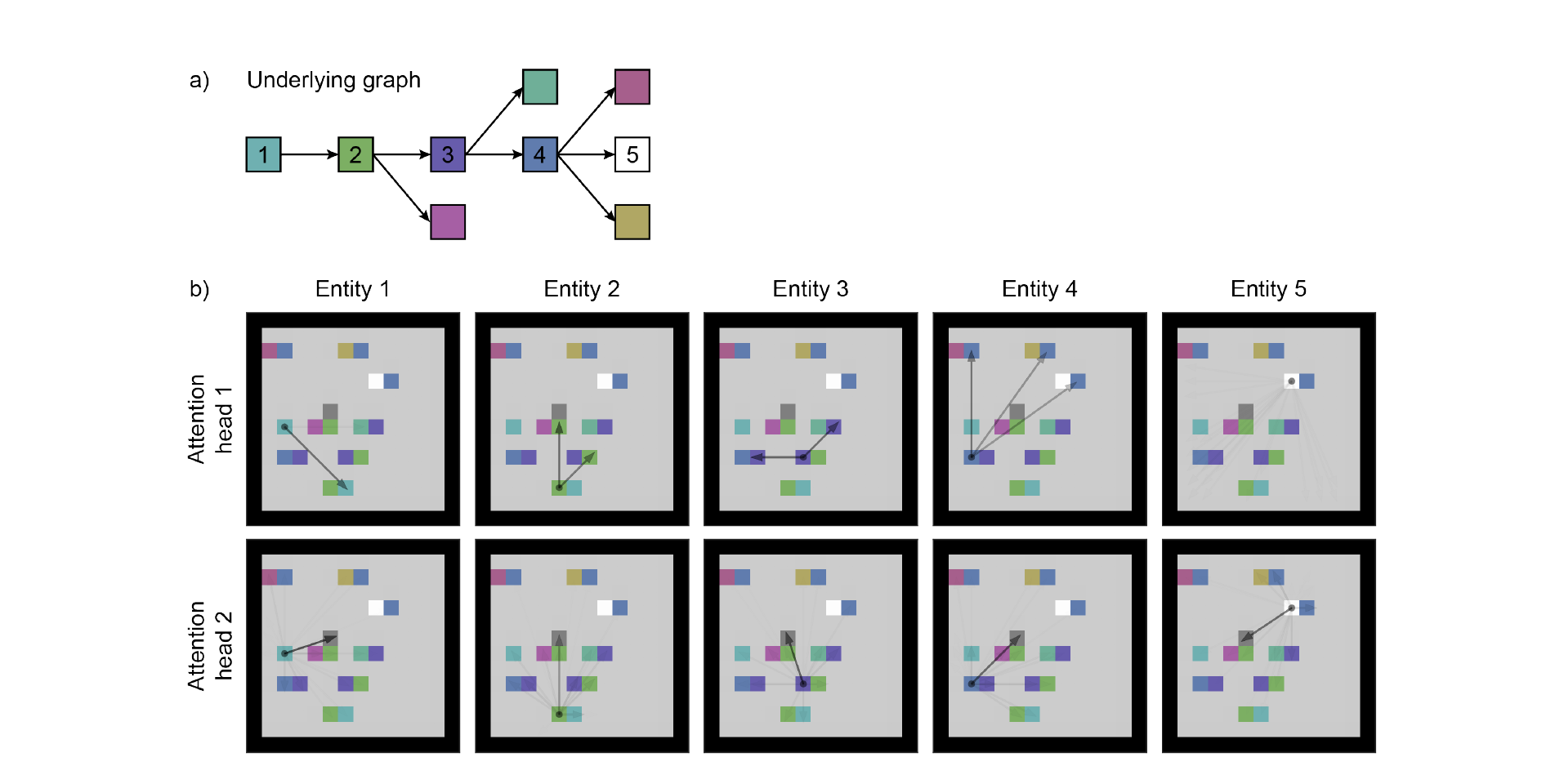## Deep Relational RL

Relational RL has not made a lot of splashes in real-world because it is easier to write a planner than learn a relational RL agent. This might be about to change with the current achievements of the graph based relational reasoning approaches. This article summarizes my understanding of the pioneering work of Vinicius Zambaldi et al. (ICLR 2019) on Deep Relational RL.

Deep RL methods have been every effective but they have poor generalization capability, especially combinatorial generalization (for eg. if the number of blocks are changed in the blocks world). Recent advances in graph network literature have achieved combinatorial generalization by learning neural network that can reason about relationship of various nodes in graphs. Since this reasoning happens pairwise, the algorithms are able to scale to varying number of objects.

In this paper, authors introduce how multi-headed dot product attention can be used to perform relational reasoning in model-free deep RL and hence achieve combinatorial generalization.

### Multi-Head Dot Product Attention (MHDPA)

This is the self attention mechanism proposed in the paper Vaswani et al. NeurIPS 2017, Attention is all you need. In that paper, the MHDPA was used on an input of word embeddings but in general it can be any form of entities. Check out the neat explanation of MHDPA by Jay Alammar here

On a very high level, attention mechanism

1. converts these entities ($X$) to Queries ($Q$), Keys ($K$) and Values ($V$) 1,
2. computes the similarity score between each query and key $QK^{T}$,
3. scales and normalizes it to a distribution: $\operatorname{Softmax}(\frac{Q.K^{T}}{\sqrt{d}})$.
4. outputs the weighted values based on this distribution: $Z = \operatorname{Softmax}(\frac{Q.K^{T}}{\sqrt{d}})\cdot V$

1. Concatenates all the attention outputs $(\mathbin\Vert_i Z_i)$
2. Transform it original $X$ dimension by multiplying it with weight matrix $W$### Summary

Zambaldi et al. proposes to use the MHDPA (with image embeddings as entities) to perform relational-reasoning while training a network for distributed A2C model. First the images from the box-world domain are processed through a convolutional neural network in the “input module”. The spatial representation learnt from the CNN is then used as embedding after concatenating $x$ and $y$ co-ordinate as additional features. MHDPA is used to perform manipulations between this entities a.k.a. relational-reasoning. Finally the multiple attention heads are aggregated by another multi-layered-perceptron $g_\theta$ (instead of the weight matrix $W$ used in Vaswani et al. 2017). Then in output module, max-pooling is performed and a FC layer converts it to actor policy $\pi$ and critic’s state-value (or advantage value) $B$.Authors mention that the use of a $g_\theta$, a non-linear MLP, in the final stage is aligned with the use of MLP in relational-network paper where a MLP is used to manipulate the relation embeddings.

Qualitative Analysis of the attention heads show that they infact learn lock-key relationship and also a relationship between agent and entities.